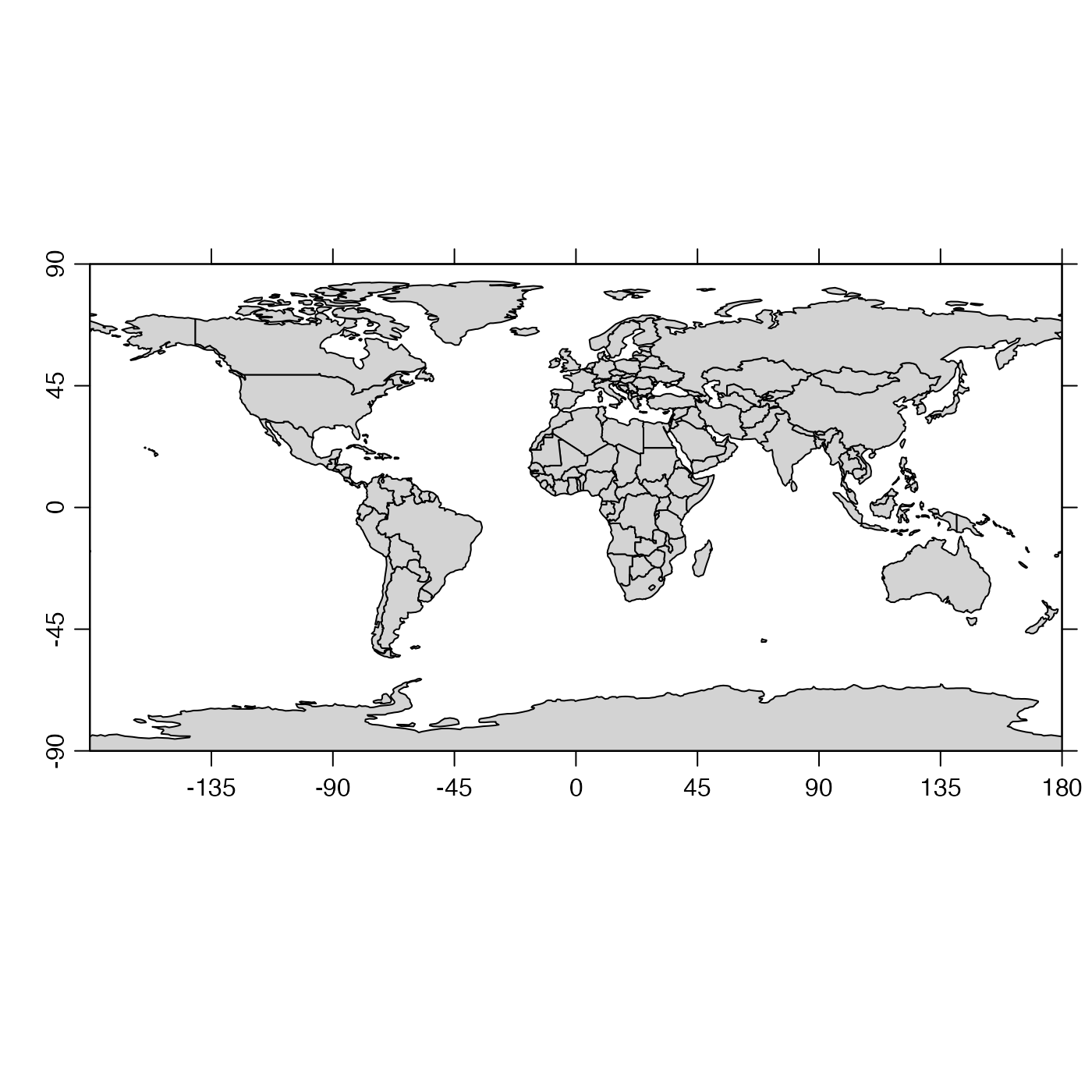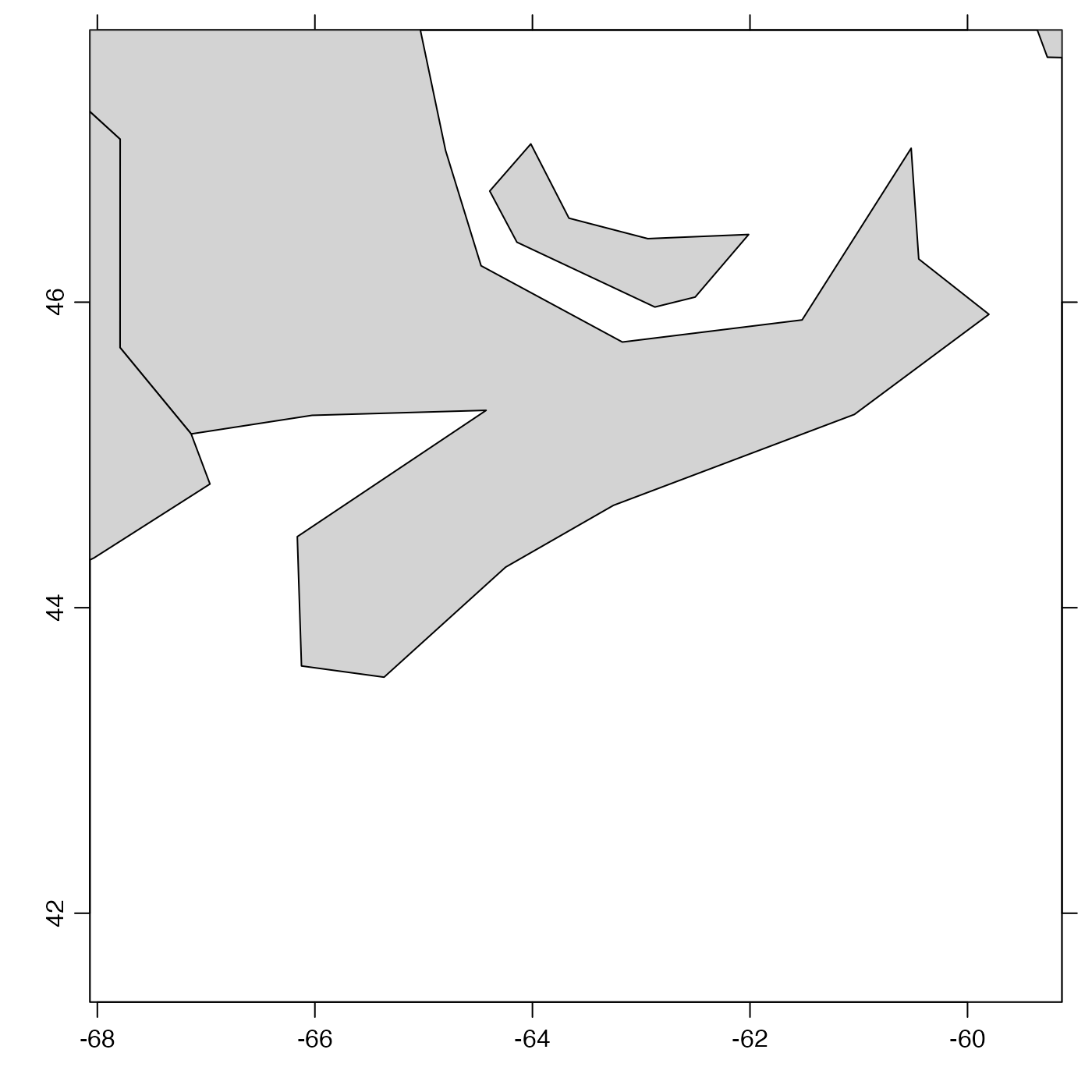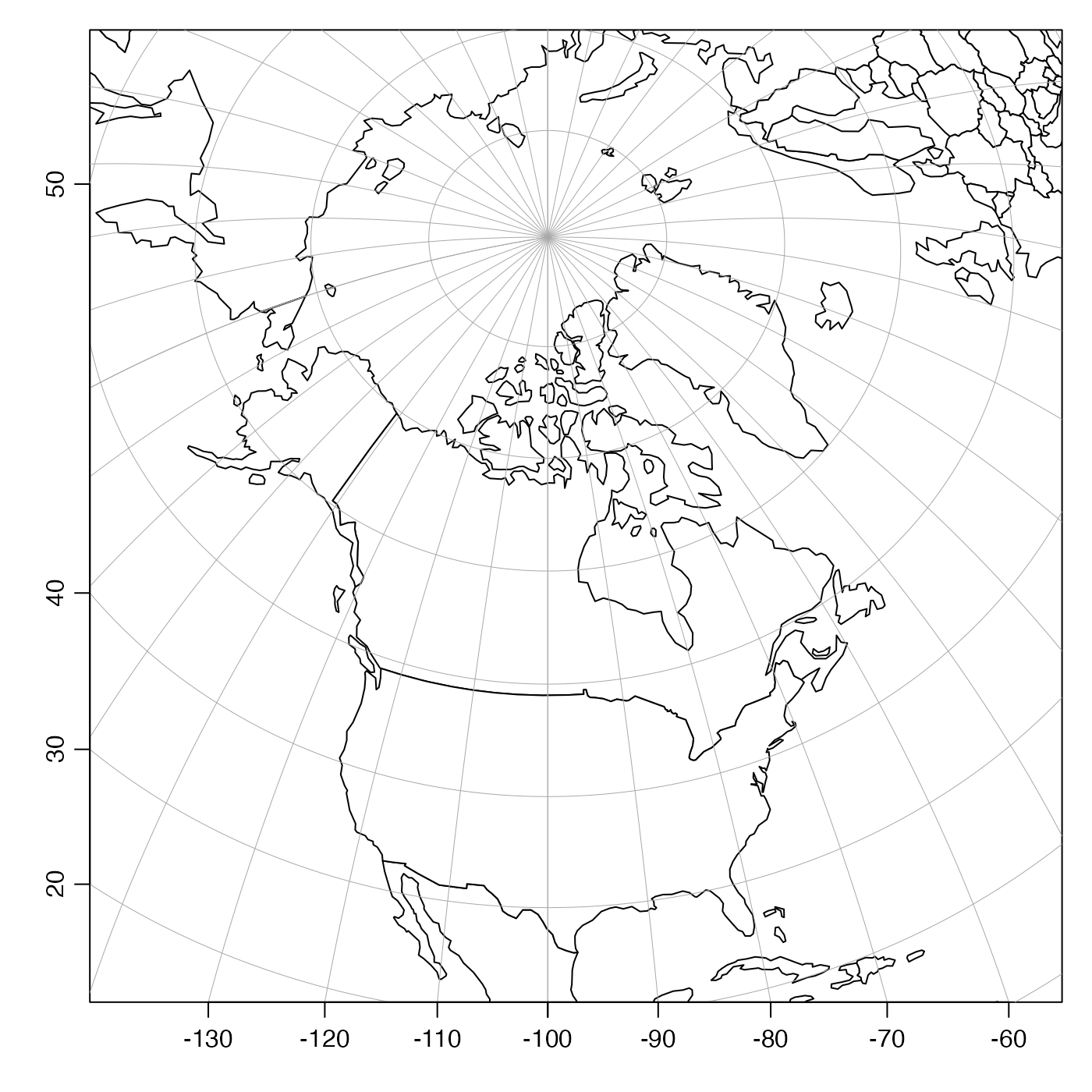This function plots a coastline. An attempt is made to fill the space of the plot, and this is done by limiting either the longitude range or the latitude range, as appropriate, by modifying the eastern or northern limit, as appropriate.

# S4 method for coastline
plot(
x,
xlab = "",
ylab = "",
showHemi = TRUE,
asp,
clongitude,
clatitude,
span,
lonlabels = TRUE,
latlabels = TRUE,
projection = NULL,
expand = 1,
mgp = getOption("oceMgp"),
mar = c(mgp + 1, mgp + 1, 1, 1),
bg,
fill,
type = "polygon",
border = NULL,
col = NULL,
axes = TRUE,
cex.axis = par("cex.axis"),
inset = FALSE,
geographical = 0,
longitudelim,
latitudelim,
debug = getOption("oceDebug"),
...
)

## Arguments

x

a coastline object.

xlab

label for x axis

ylab

label for y axis

showHemi

logical indicating whether to show the hemisphere in axis tick labels.

asp

Aspect ratio for plot. The default is for plot,coastline-method to set the aspect ratio to give natural latitude-longitude scaling somewhere near the centre latitude on the plot. Often, it makes sense to set asp yourself, e.g. to get correct shapes at 45N, use asp=1/cos(45*pi/180). Note that the land mass is not symmetric about the equator, so to get good world views you should set asp=1 or set ylim to be symmetric about zero. Any given value of asp is ignored, if clongitude and clatitude are given (or if the latter two are inferred from projection.

clongitude, clatitude

optional center latitude of map, in decimal degrees. If both clongitude and clatitude are provided, or alternatively if they can be inferred from substrings +lon_0 and +lat_0 in projection, then any provided value of asp is ignored, and instead the plot aspect ratio is computed based on the center latitude. If clongitude and clatitude are known, then span must also be provided, and in this case, it is not permitted to also specify longitudelim and latitudelim.

span

optional suggested diagonal span of the plot, in kilometers. The plotted span is usually close to the suggestion, although the details depend on the plot aspect ratio and other factors, so some adjustment may be required to fine-tune a plot. A value for span must be supplied, if clongitude and clatitude are supplied (or inferred from projection).

lonlabels, latlabels

optional vectors of longitude and latitude to label on the sides of plot, passed to mapPlot() to control axis labelling, for plots done with map projections (i.e. for cases in which projection is not NULL).

projection

optional map projection to use (see the mapPlot() argument of the same name). If set to FALSE then no projection is used, and the data are plotted in a cartesion frame, with aspect ratio set to reduce distortion near the middle of the plot. This option is useful if the coastline produces spurious horizontal lines owing to islands crossing the plot edges (a problem that plagues map projections). If projection is not set, a Mercator projection is used for latitudes below about 70 degrees, as if projection="+proj=merc" had been supplied, or a Stereopolar one is used as if projection="+proj=stere". Otherwise, projection must be a character string identifying a projection accepted by mapPlot().

expand

numerical factor for the expansion of plot limits, showing area outside the plot, e.g. if showing a ship track as a coastline, and then an actual coastline to show the ocean boundary. The value of expand is ignored if either xlim or ylim is given.

mgp

3-element numerical vector to use for par("mgp"), and also for par(mar), computed from this. The default is tighter than the R default, in order to use more space for the data and less for the axes.

mar

value to be used with par("mar").

bg

optional color to be used for the background of the map. This comes in handy for drawing insets (see “details”).

fill

a legacy parameter that will be permitted only temporarily; see “History”.

type

indication of type; may be "polygon", for a filled polygon, "p" for points, "l" for line segments, or "o" for points overlain with line segments. See color for a note on how the the value of type alters the meaning of the color argument.

border

color used to indicate land (if type="polygon") or the coastline and international borders (if type="l").

col

either the color for filling polygons (if type="polygon") or the color of the points and line segments (if type="p", type="l", or type="o").

axes

boolean, set to TRUE to plot axes.

cex.axis

value for axis font size factor.

boolean, set to TRUE to draw the coastline on an existing plot. Note that this retains the aspect ratio of that existing plot, so it is important to set that correctly, e.g. with asp=1/cos(lat * pi / 180), where clat is the central latitude of the plot.

inset

set to TRUE for use within plotInset(). The effect is to prevent the present function from adjusting margins, which is necessary because margin adjustment is the basis for the method used by plotInset().

geographical

flag indicating the style of axes. With geographical=0, the axes are conventional, with decimal degrees as the unit, and negative signs indicating the southern and western hemispheres. With geographical=1, the signs are dropped, with axis values being in decreasing order within the southern and western hemispheres. With geographical=2, the signs are dropped and the axes are labelled with degrees, minutes and seconds, as appropriate, and hemispheres are indicated with letters. With geographical=3, things are the same as for geographical=2, but the hemisphere indication is omitted. Finally, with geographical=4, unsigned numbers are used, followed by letters N in the northern hemisphere, S in the southern, E in the eastern, and W in the western.

longitudelim

this and latitudelim provide a second way to suggest plot ranges. Note that these may not be supplied if clongitude, clatitude and span are given.

latitudelim

see longitudelim.

debug

set to TRUE to get debugging information during processing.

...

optional arguments passed to plotting functions. For example, set yaxp=c(-90,90,4) for a plot extending from pole to pole.

None.

## Details

If longitudelim, latitudelim and projection are all given, then these arguments are passed to mapPlot() to produce the plot. (The call uses bg for col, and uses col, fill and border directly.) If the results need further customization, users should use mapPlot() directly.

If projection is provided without longitudelim or latitudelim, then mapPlot() is still called, but longitudelim and latitudelim are computed from clongitude, clatitude and span.

If projection is not provided, much simpler plots are produced. These are Cartesian, with aspect ratio set to minimize shape distortion at the central latitude. Although these are crude, they have the benefit of always working, which cannot be said of true map projections, which can be problematic in various ways owing to difficulties in inverting projection calculations.

To get an inset map inside another map, draw the first map, do par(new=TRUE), and then call plot,coastline-method() with a value of mar that moves the inset plot to a desired location on the existing plot, and with bg="white".

## History

Until February, 2016, plot,coastline-method relied on a now-defunct argument fill to control colors; col is to be used now, instead.

The documentation for the coastline class explains the structure of coastline objects, and also outlines the other functions dealing with them.

Other functions that plot oce data: download.amsr(), plot,adp-method, plot,adv-method, plot,amsr-method, plot,argo-method, plot,bremen-method, plot,cm-method, plot,ctd-method, plot,gps-method, plot,ladp-method, plot,landsat-method, plot,lisst-method, plot,lobo-method, plot,met-method, plot,odf-method, plot,rsk-method, plot,satellite-method, plot,sealevel-method, plot,section-method, plot,tidem-method, plot,topo-method, plot,windrose-method, plot,xbt-method, plotProfile(), plotScan(), plotTS(), tidem-class

Other things related to coastline data: [[,coastline-method, [[<-,coastline-method, as.coastline(), coastline-class, coastlineBest(), coastlineCut(), coastlineWorld, download.coastline(), read.coastline.openstreetmap(), read.coastline.shapefile(), subset,coastline-method, summary,coastline-method

Dan Kelley

## Examples

# \donttest{
library(oce)
par(mar=c(2, 2, 1, 1))
data(coastlineWorld)
plot(coastlineWorld)plot(coastlineWorld, clongitude=-63.6, clatitude=44.6, span=1000)